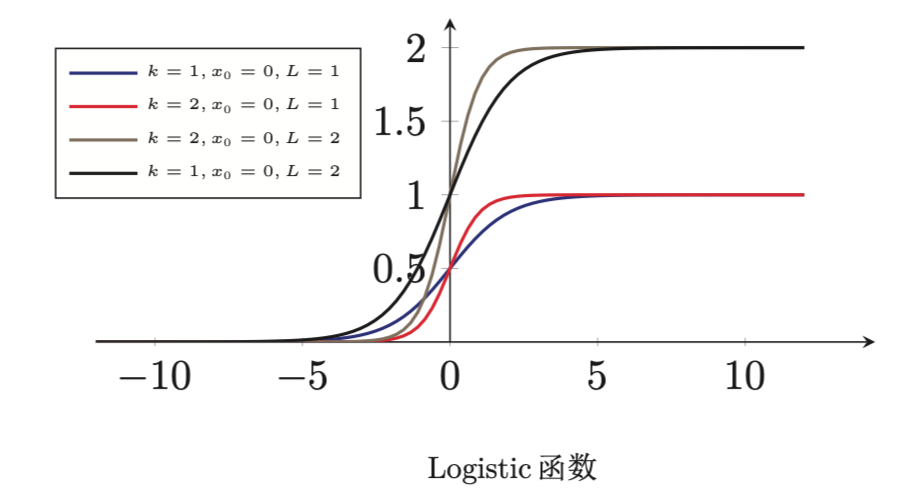【算法工程师的数学基础】系列将会从线性代数、微积分、数值优化、概率论、信息论五个方面进行介绍，感兴趣的欢迎关注【搜索与推荐Wiki】公众号，获得最新文章。

《算法工程师的数学基础》已更新：

### 导数法则

#### 加减法则

$y = f(x), z=g(x)$ 则：

#### 乘法法则

(1) 若 $x \in R^p, y= f(x) \in R^q, z = g(x) \in R^q$ ，则：

(2) 若 $x \in R^p, y= f(x) \in R^s, z = g(x) \in R^t, A \in R^{s *t}$ 和 $x$无关，则：

(3)若 $x \in R^p, y= f(x) \in R, z = g(x) \in R^p$ ，则：

#### 链式法则

(1) 若 $x \in R^p, y= g(x) \in R^s, z = f(y) \in R^t$ ，则：

(2)若 $X \in R^{pq}$为矩阵, $Y= g(x) \in R^{st}, z = f(Y) \in R$ ，则：

(3)若 $X \in R^{p*q}$为矩阵, $y= g(x) \in R^s, z = f(Y) \in R$ ，则

(4)若 $x \in R, u= u(x) \in R^p, g = g(u) \in R^q$ ，则：

(4)的文字部分，$u = u(x)$中左边的$u$表示向量， $g=g(u)$左边的$g$表示向量，$g(u)$表示函数，$u$表示向量

### 常见函数的导数

#### logistic函数

Logistic函数是一种常用的S形函数，是比利时数学家Pierrs Francois Verhulst 在1844-1845年研究种群数量的增长模型时提出命名的，最初作为一种生态学模型。

Logistic的函数定义为：#### softmax函数

softmax函数是将多个标量映射为一个概率分布。

### 基本求导函数法则与导数公式

#### 双曲函数和反双曲函数的导数

（1）双曲正弦函数和反双曲正弦函数

（2）双曲余弦函数和反双曲余弦函数

（3）双曲正切函数和反双曲正切函数

• 双曲余切函数和反双曲余切函数
• 双曲正割函数和双曲正割函数
• 双曲余割函数和双曲余割函数

【技术服务】，详情点击查看： https://mp.weixin.qq.com/s/PtX9ukKRBmazAWARprGIAg